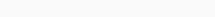# Math Puzzles Puzzle 66 Answer AndroidMath puzzle game level 66 solution with detailed hints and explanation, Scroll below to find out.

Math puzzles increase your logical thinking. The objective of the game is to find patterns hidden in the puzzle in order to arrive at the solution. Challenge yourself with different levels of math puzzles and stretch the limits of your intelligence.

### Math Puzzles Puzzle 66:

18,24,32

12,14,16

3, ?, 4

72, 112, 128Solution/Hint:

For each column (Last number/First number) = (Second number/Third Number)

If there is any Doubt or you are unable to understand the solution, then please let me know in comments, I will surely help you.

### 3 thoughts on “Math Puzzles Game Level 66 Answer with Solution”

•May 1, 2019 at 12:49

Your solution seems not working on the second column. It should be more like first number / (last number / second number) = third number

•May 1, 2019 at 13:01
•